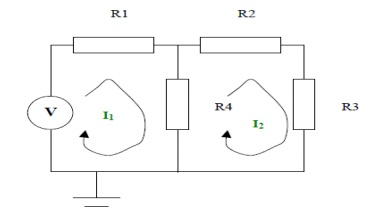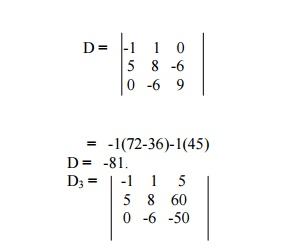Home | | Circuit Theory | Mesh Analysis

# Mesh Analysis

This is an alternative structured approach to solving the circuit and is based on calculating mesh currents.

MESH ANALYSIS:

This is an alternative structured approach to solving the circuit and is based on calculating mesh currents. A similar approach to the node situation is used. A set of equations (based on KVL for each mesh) is formed and the equations are solved for unknown values. As many equations are needed as unknown mesh currents exist.

Step 1: Identify the mesh currents

Step 2: Determine which mesh currents are known

Step 2: Write equation for each mesh using KVL and that includes the mesh currents Step 3: Solve the equations

Step 1:

The mesh currents are as shown in the diagram on the next page

Step 2:

Neither of the mesh currents is knownStep 3:

KVL can be applied to the left hand side loop. This states the voltages around the loop sum to zero.

When writing down the voltages across each resistor equations are the mesh currents.

I1R1 + (I1 - I2) R4 - V = 0

KVL can be applied to the right hand side loop. This states the voltages around the loop sum to

zero. When writing down the voltages across ea the equations are the mesh currents.

I2R2 + I2R3 + (I2 - I1) R4 = 0

Step 4:

Solving the equations we getThe individual branch currents can be obtained from the these mesh currents and the node voltages can also be calculated using this information. For example:Problem 1:

Use mesh-current analysis to determine the current flowing in (a the 1╬® resistance of the d.c. circuit shown inThe mesh currents I1, I2 and I3 are shown in Figure

Using         KirchhoffŌĆÖs   voltage   law:

For loop 1, (3 + 5) I1 ŌłÆI2 = 4   ŌĆ”ŌĆ”ŌĆ”ŌĆ”ŌĆ”ŌĆ”ŌĆ”ŌĆ”ŌĆ”ŌĆ”ŌĆ”ŌĆ”ŌĆ”ŌĆ”ŌĆ”ŌĆ”ŌĆ”ŌĆ”ŌĆ”ŌĆ”ŌĆ”ŌĆ”(1)

For loop 2, (4 + 1 + 6 + 5) I2 ŌłÆ(5) I1 ŌłÆ(1) I3 = 0ŌĆ”ŌĆ”ŌĆ”ŌĆ”ŌĆ”ŌĆ”ŌĆ”ŌĆ”ŌĆ”ŌĆ”ŌĆ”ŌĆ”ŌĆ”ŌĆ”.(2)

For loop 3, (1 + 8) I3 ŌłÆ(1) I2 = 5ŌłÆ ŌĆ”ŌĆ”ŌĆ”ŌĆ”ŌĆ”ŌĆ”ŌĆ”ŌĆ”ŌĆ”ŌĆ”ŌĆ”ŌĆ”ŌĆ”ŌĆ”ŌĆ”ŌĆ”ŌĆ”ŌĆ”ŌĆ”ŌĆ”(3)

Thus

8I1 ŌłÆ5I2 ŌłÆ4 =0

ŌłÆ5I1 + 16I2 ŌłÆI3 =0

ŌłÆI2 + 9I3 + 5 =0(a) Current in the 5 ╬®resistance = I1 ŌłÆI2

= 0.595 ŌłÆ0.152

= 0.44A

(b) Current in the 1 ╬®resistance = I2 ŌłÆI3

= 0.152 ŌłÆ(ŌłÆ0.539)

= 0.69A

Problem 2: For the a.c. network shown in Figure determine, using mesh-current analysis, (a) the mesh currents I1 and I2 (b) the current flowing in the capacitor, and (c) the active power delivered by the 100ŌłĀ0ŌŚ”V voltage source.(a) For the first loop

(5ŌłÆj4) I1 ŌłÆ(ŌłÆj4I2) =100ŌłĀ0ŌŚ”ŌĆ”ŌĆ”ŌĆ”ŌĆ”ŌĆ”ŌĆ”ŌĆ”ŌĆ”ŌĆ”ŌĆ”ŌĆ”ŌĆ”ŌĆ”ŌĆ”ŌĆ”ŌĆ”ŌĆ”ŌĆ”ŌĆ”ŌĆ”(1) For the second loop

(4+j3ŌłÆj4)I2 ŌłÆ(ŌłÆj4I1) =0   ŌĆ”ŌĆ”ŌĆ”ŌĆ”ŌĆ”ŌĆ”ŌĆ”ŌĆ”ŌĆ”ŌĆ”ŌĆ”ŌĆ”ŌĆ”ŌĆ”ŌĆ”ŌĆ”ŌĆ”ŌĆ”ŌĆ”ŌĆ”ŌĆ”   (2)

Rewriting equations (1) and (2) gives:

(5 ŌłÆj4)I1 + j4I2 ŌłÆ100 =0

j4I1 + (4 ŌłÆj) I2 + 0 =0 Thus, using determinants,Thus total power dissipated = 579.97 + 436.81 = 1016.8W = 1020W

Problem 3: Calculate   current   through  -6╬® resistance   uCase(1): Consider loop ABGH ; Apply KVL .D3 = 6(220) +4(-80) +10(-24)

D3 = 760

I1 = D1/D = 260/284 = 0.915A

I2 = D2/D = -320/284 = -1.1267A I3 = D3/D = 760/284 = 2.676A

Current through   6╬® 2 +Iresistance3    =   I

= -1.1267+2.676 = 1.55A

Problem 4: Find the current through branch a-b using mesh analysis.Solution:

Consider loops

Loop HADE  - > 5I1+2I2+6(I2-I3) = 60

5I1+8I2-6I3 = 60 ------------ (1)

Loop ABCDA  - > 3I3+6(I3-I2) = -50

3I3+6I3-6I2 = -50

9I3-6I2 = -50---------- (2)

I2-I1 = 5A ------------------------- (3)

From (1), (2) & (3).=     -1(-400+360)-(-250) +5(-30)

=         40+250-150

D3 =   140.

I3 = D3/D = 140/-81 = -1.7283

The current through branch ab is 1.7283A which is flowing from b to a.

Study Material, Lecturing Notes, Assignment, Reference, Wiki description explanation, brief detail
Electrical and electronics : Circuit Theory : Mesh Analysis |